# LARGE Function

Returns the numeric value sorted by value in descending order

## What is the Excel LARGE Function?

The Excel LARGE Function is categorized under Excel Statistical functions. The function will return the k largest value, sorted by value in descending order.

As a financial analyst, the LARGE function can be used to sort information provided and find the maximum value, such as share price or rate of return for a portfolio.

### Formula

=LARGE(array,k)

The formula uses the following arguments:

1. Array (required argument) – This is the array or range of data for which we wish to determine the largest value.
2. K (required argument) – This is an integer that specifies the position from the largest value in the array or range of cells of data to return.

### How to use the LARGE Function in Excel?

To understand the uses of the LARGE function, let’s consider a few examples:

#### Example 1

Suppose we are given the following data: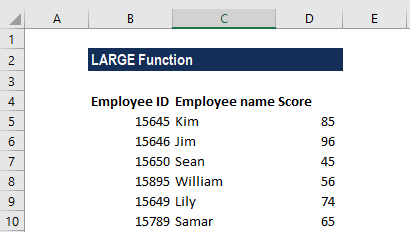Here, we wish to find out the names of employees with the top 3 scores. The formulas to use are below. For the highest score: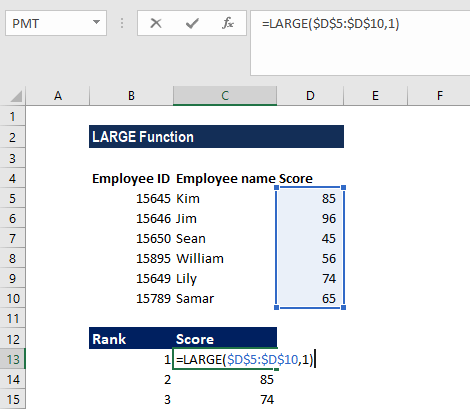And the second-highest score: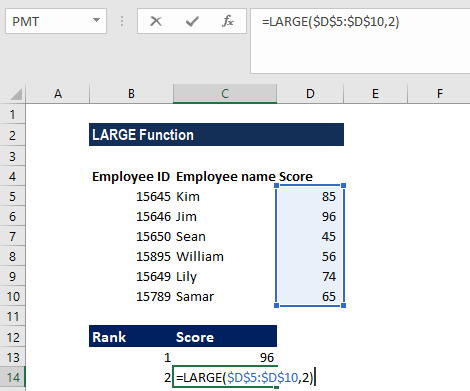For the third place: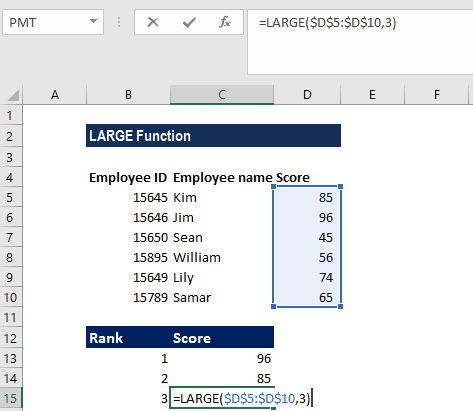We get the results below: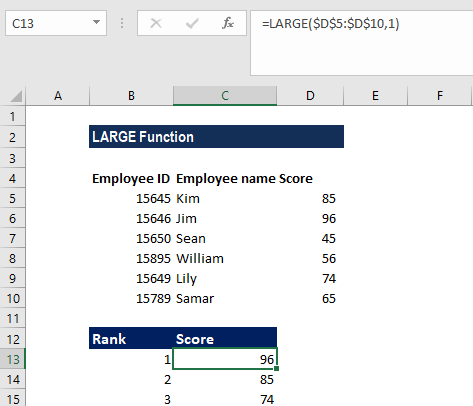#### Example 2

Suppose we are given the following data: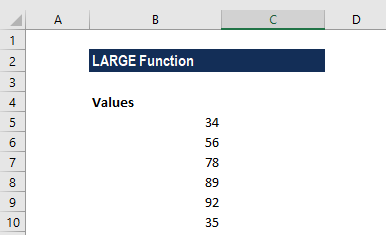We can sum the top values in a range. The formula used for the three highest values is: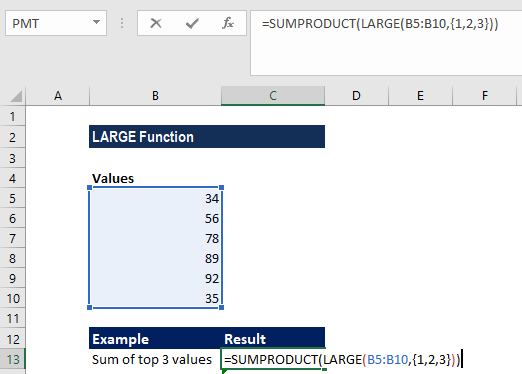We get the result below: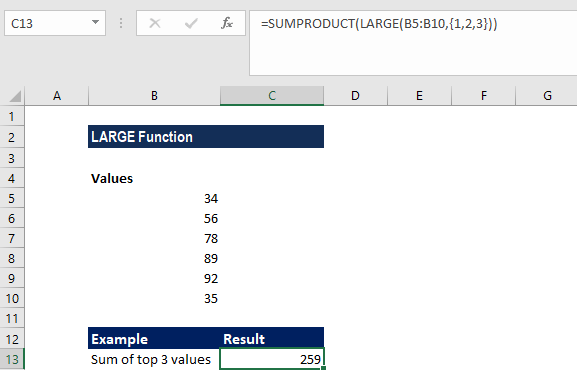Here, we used the formula that was based on the LARGE function that was wrapped inside the SUMPRODUCT function.

LARGE will return an array as we provided an array constant. SUMPRODUCT then added up the results received to give the desired information.

Similarly, we find the sum of the top four values of the array, as shown below: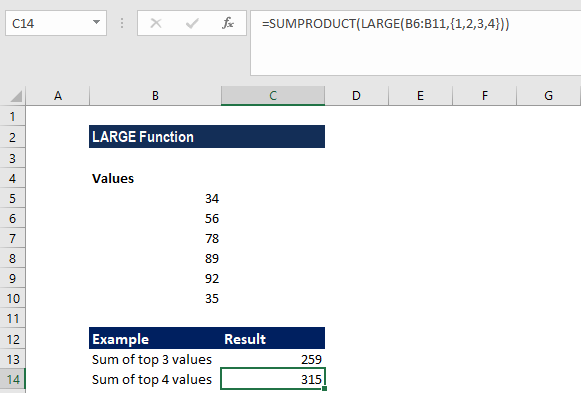### Notes about the Excel LARGE Function

1. #VALUE! error – Occurs when the supplied k argument is non-numeric.
2. #NUM error – Occurs when:
• The array provided is empty.
• When the value of the k argument is less than 1 or greater than the number of values in the given array.

Thanks for reading CFI’s guide to important Excel functions! By taking the time to learn and master these Excel functions, you’ll significantly speed up your financial analysis. To learn more, check out these additional CFI resources:

• Excel Functions for Finance